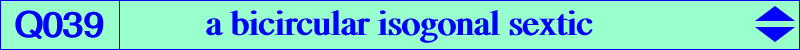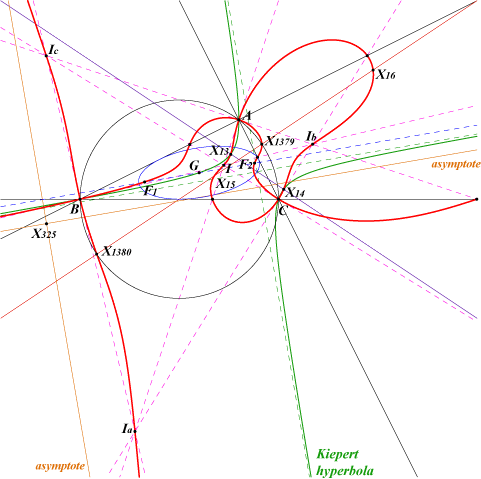too complicated to be written here. Click on the link to download a text file.See another form below.X(1), X(13), X(14), X(15), X(16), X(1379), X(1380), X(3413), X(3414) Ia, Ib, Ic : excenters 6 feet of bisectors anti-points, see Table 77 F1, F2 : foci of the inscribed Steiner ellipse imaginary foci of the Brocard ellipse i.e. common points of the Brocard axis and the Kiepert hyperbolaQ039 is the locus of point P whose pedal triangle has a Brocard line passing through P. For the Euler line, see Q002. It is also the locus of P such that the circum-strophoid with node P is a K0, i.e. has no term in xyz in its equation. See CL038. The points A, B, C are nodes with nodal tangents the bisectors. Q039 has two real asymptotes which are the parallels to those of the Kiepert hyperbola at X(325), the isotomic conjugate of the Tarry point X(98). See the related sextics Q075 and Q076. See also Panchapakesan isogonal sextics at Q170. The equation of Q039 can be written under the form : 4xyz ∑(a2 SA-SB SC) x (c2y2- b2z2) + ∏(c2y2- b2z2) = 0, where ∑(a2 SA-SB SC) x (c2y2- b2z2) = 0 is the equation of the Darboux cubic K004 and ∏(c2y2- b2z2) = 0 is that of the six bisectors of ABC. It follows that the tangents at the in/excenters to Q039 pass through the de Longchamps point X(20) of ABC.